Next: Theorems Up: Fibonacci Numbers (last year) Previous: Fibonacci Numbers (last year)   Contents

## Definitions

• The Fibonacci sequence (the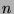th term of which is denoted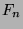) is defined recursively by the following statements:
•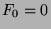.
•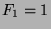.
•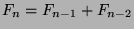,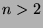.
•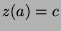means that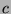has the following properties:
•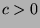.
•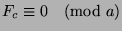.
•is the least positive integer satisfying these properties.
•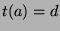means that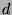has the following properties:
•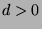.
•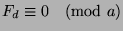.
•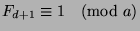.
•is the least positive integer satisfying these properties.
Note: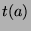is then the function that gives the period of the Fibonacci numbers in modulo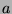.
•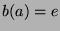means that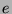has the following properties:
•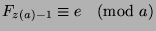.

Gregory Stoll 2000-04-08